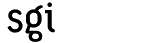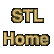# Introduction to the Standard Template Library

The Standard Template Library, or STL, is a C++ library of container classes, algorithms, and iterators; it provides many of the basic algorithms and data structures of computer science. The STL is a generic library, meaning that its components are heavily parameterized: almost every component in the STL is a template. You should make sure that you understand how templates work in C++ before you use the STL.

## Containers and algorithms

Like many class libraries, the STL includes container classes: classes whose purpose is to contain other objects. The STL includes the classes vector, list, deque, set, multiset, map, multimap, hash_set, hash_multiset, hash_map, and hash_multimap. Each of these classes is a template, and can be instantiated to contain any type of object. You can, for example, use a vector<int> in much the same way as you would use an ordinary C array, except that vector eliminates the chore of managing dynamic memory allocation by hand.

```      vector<int> v(3);            // Declare a vector of 3 elements.
v = 7;
v = v + 3;
v = v + v;          // v == 7, v == 10, v == 17
```

The STL also includes a large collection of algorithms that manipulate the data stored in containers. You can reverse the order of elements in a vector, for example, by using the reverse algorithm.

```      reverse(v.begin(), v.end()); // v == 17, v == 10, v == 7
```

There are two important points to notice about this call to reverse. First, it is a global function, not a member function. Second, it takes two arguments rather than one: it operates on a range of elements, rather than on a container. In this particular case the range happens to be the entire container v.

The reason for both of these facts is the same: reverse, like other STL algorithms, is decoupled from the STL container classes. This means that reverse can be used not only to reverse elements in vectors, but also to reverse elements in lists, and even elements in C arrays. The following program is also valid.

```      double A = { 1.2, 1.3, 1.4, 1.5, 1.6, 1.7 };
reverse(A, A + 6);
for (int i = 0; i < 6; ++i)
cout << "A[" << i << "] = " << A[i];
```

This example uses a range, just like the example of reversing a vector: the first argument to reverse is a pointer to the beginning of the range, and the second argument points one element past the end of the range. This range is denoted [A, A + 6); the asymmetrical notation is a reminder that the two endpoints are different, that the first is the beginning of the range and the second is one past the end of the range.

## Iterators

In the example of reversing a C array, the arguments to reverse are clearly of type double*. What are the arguments to reverse if you are reversing a vector, though, or a list? That is, what exactly does reverse declare its arguments to be, and what exactly do v.begin() and v.end() return?

The answer is that the arguments to reverse are iterators, which are a generalization of pointers. Pointers themselves are iterators, which is why it is possible to reverse the elements of a C array. Similarly, vector declares the nested types iterator and const_iterator. In the example above, the type returned by v.begin() and v.end() is vector<int>::iterator. There are also some iterators, such as istream_iterator and ostream_iterator, that aren't associated with containers at all.

Iterators are the mechanism that makes it possible to decouple algorithms from containers: algorithms are templates, and are parameterized by the type of iterator, so they are not restricted to a single type of container. Consider, for example, how to write an algorithm that performs linear search through a range. This is the STL's find algorithm.

```      template <class InputIterator, class T>
InputIterator find(InputIterator first, InputIterator last, const T& value) {
while (first != last && *first != value) ++first;
return first;
}
```

Find takes three arguments: two iterators that define a range, and a value to search for in that range. It examines each iterator in the range [first, last), proceeding from the beginning to the end, and stops either when it finds an iterator that points to value or when it reaches the end of the range.

First and last are declared to be of type InputIterator, and InputIterator is a template parameter. That is, there isn't actually any type called InputIterator: when you call find, the compiler substitutes the actual type of the arguments for the formal type parameters InputIterator and T. If the first two arguments to find are of type int* and the third is of type int, then it is as if you had called the following function.

```      int* find(int* first, int* last, const int& value) {
while (first != last && *first != value) ++first;
return first;
}
```

## Concepts and Modeling

One very important question to ask about any template function, not just about STL algorithms, is what the set of types is that may correctly be substituted for the formal template parameters. Clearly, for example, int* or double* may be substituted for find's formal template parameter InputIterator. Equally clearly, int or double may not: find uses the expression *first, and the dereference operator makes no sense for an object of type int or of type double. The basic answer, then, is that find implicitly defines a set of requirements on types, and that it may be instantiated with any type that satisfies those requirements. Whatever type is substituted for InputIterator must provide certain operations: it must be possible to compare two objects of that type for equality, it must be possible to increment an object of that type, it must be possible to dereference an object of that type to obtain the object that it points to, and so on.

Find isn't the only STL algorithm that has such a set of requirements; the arguments to for_each and count, and other algorithms, must satisfy the same requirements. These requirements are sufficiently important that we give them a name: we call such a set of type requirements a concept, and we call this particular concept Input Iterator. We say that a type conforms to a concept, or that it is a model of a concept, if it satisfies all of those requirements. We say that int* is a model of Input Iterator because int* provides all of the operations that are specified by the Input Iterator requirements.

Concepts are not a part of the C++ language; there is no way to declare a concept in a program, or to declare that a particular type is a model of a concept. Nevertheless, concepts are an extremely important part of the STL. Using concepts makes it possible to write programs that cleanly separate interface from implementation: the author of find only has to consider the interface specified by the concept Input Iterator, rather than the implementation of every possible type that conforms to that concept. Similarly, if you want to use find, you need only to ensure that the arguments you pass to it are models of Input Iterator. This is the reason why find and reverse can be used with lists, vectors, C arrays, and many other types: programming in terms of concepts, rather than in terms of specific types, makes it possible to reuse software components and to combine components together.

## Refinement

Input Iterator is, in fact, a rather weak concept: that is, it imposes very few requirements. An Input Iterator must support a subset of pointer arithmetic (it must be possible to increment an Input Iterator using prefix and postfix operator++), but need not support all operations of pointer arithmetic. This is sufficient for find, but some other algorithms require that their arguments satisfy additional requirements. Reverse, for example, must be able to decrement its arguments as well as increment them; it uses the expression --last. In terms of concepts, we say that reverse's arguments must be models of Bidirectional Iterator rather than Input Iterator.

The Bidirectional Iterator concept is very similar to the Input Iterator concept: it simply imposes some additional requirements. The types that are models of Bidirectional Iterator are a subset of the types that are models of Input Iterator: every type that is a model of Bidirectional Iterator is also a model of Input Iterator. Int*, for example, is both a model of Bidirectional Iterator and a model of Input Iterator, but istream_iterator, is only a model of Input Iterator: it does not conform to the more stringent Bidirectional Iterator requirements.

We describe the relationship between Input Iterator and Bidirectional Iterator by saying that Bidirectional Iterator is a refinement of Input Iterator. Refinement of concepts is very much like inheritance of C++ classes; the main reason we use a different word, instead of just calling it "inheritance", is to emphasize that refinement applies to concepts rather than to actual types.

There are actually three more iterator concepts in addition to the two that we have already discussed: the five iterator concepts are Output Iterator, Input Iterator, Forward Iterator, Bidirectional Iterator, and Random Access Iterator; Forward Iterator is a refinement of Input Iterator, Bidirectional Iterator is a refinement of Forward Iterator, and Random Access Iterator is a refinement of Bidirectional Iterator. (Output Iterator is related to the other four concepts, but it is not part of the hierarchy of refinement: it is not a refinement of any of the other iterator concepts, and none of the other iterator concepts are refinements of it.) The Iterator Overview has more information about iterators in general.

Container classes, like iterators, are organized into a hierarchy of concepts. All containers are models of the concept Container; more refined concepts, such as Sequence and Associative Container, describe specific types of containers.

## Other parts of the STL

If you understand algorithms, iterators, and containers, then you understand almost everything there is to know about the STL. The STL does, however, include several other types of components.

First, the STL includes several utilities: very basic concepts and functions that are used in many different parts of the library. The concept Assignable, for example, describes types that have assignment operators and copy constructors; almost all STL classes are models of Assignable, and almost all STL algorithms require their arguments to be models of Assignable.

Second, the STL includes some low-level mechanisms for allocating and deallocating memory. Allocators are very specialized, and you can safely ignore them for almost all purposes.

Finally, the STL includes a large collection of function objects, also known as functors. Just as iterators are a generalization of pointers, function objects are a generalization of functions: a function object is anything that you can call using the ordinary function call syntax. There are several different concepts relating to function objects, including Unary Function (a function object that takes a single argument, i.e. one that is called as f(x)) and Binary Function (a function object that takes two arguments, i.e. one that is called as f(x, y)). Function objects are an important part of generic programming because they allow abstraction not only over the types of objects, but also over the operations that are being performed.